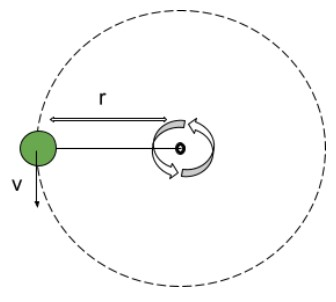# Centripetal Acceleration Calculator

Enter the values of radius and velocity in the centripetal acceleration calculator to calculate centripetal acceleration.

Formula:
a = v2r

Give Us Feedback

Centripetal acceleration calculator helps you in calculating centripetal acceleration if the velocity of the body and radius of the circle is given. It will give you a step-by-step process as well.

This calculator can also be used to calculate radius and velocity.

## What is centripetal acceleration?Due to its direction, centripetal acceleration is also known as radial acceleration. The definition of centripetal acceleration is stated below.

“Centripetal acceleration is a property of the motion of a body traversing a circular path.”

The acceleration is directed radially toward the center of the circle and has a magnitude equal to the square of the body’s speed along the curve divided by the distance from the center of the circle to the moving body.

## Centripetal acceleration formula

The equation used for calculating the value of centripetal acceleration:

ac = v2/r

In this equation:

V is centripetal velocity,

## How to calculate centripetal acceleration?

Example:

A car is moving in a circle of a radius of 20 meters at the velocity of 10 meters per second. What is the centripetal acceleration of the car?

Solution:

Step 1: Identify the values:

V = 10 m/s

r = 20 m

Step 2: Put the values in the formula of centripetal acceleration:

ac = v2/r

ac = 102/20

= 5 m/s2

### Math Tools